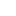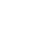# Solution preparation of different concentrations

Solution preparation of different concentrations

#### Solution:

Homogeneous mixture of two or more substances or components, which can exist in any phase, is called a solution. A solution consists of two components.

• Solvent
• Solute

#### Solvent:

The substance which is present in large quantity in the solution in same phase is called solvent. Solvent has greater affinity for the solute particles.

#### Solute:

The substance or component of solution that is present in lower concentration in same phase is known as solute. Solvent has a great force of attraction for solute particles.

For example:

A saline solution is prepared by mixing the salt (NaCl) as a solute and water as a solvent.

#### Solubility:

The amount of solute which is dissolved in a solvent at specific temperature is known as solubility. Solubility is a temperature dependent phenomenon.

#### Distinctive properties of solutions:

A chemical solution has some important properties which are:

• A solution is composed of one phase while solute and solvent may present in different phases
• A solution is homogeneous mixture of two or more than two components may be of phase or different phase
• A naked eye cannot differentiate or see the solution particles
• Light beam is not scattered by particles of the clear solution

#### Types of solution:

On the basis of concentrations of components there are following types of solution.

• Concentrated solution
• Dilute solution

#### Concentrated solution:

The solution in which there is relatively large amount of a substance is known as concentrated solution.

#### Dilute solution:

The solution which contains relatively less amount of substance or solute is called dilute solution.

For example:

5 M solution of NaCl in 100 ml is dilute than the respective 10 M solution

#### Colloidal solution:

A solution which contains particles of sizes ranging from 1 to 1000 nanometers in diameter which remain equally distributed in the solution is called as colloidal solution. In this the substance remains in dispersed form.

There are some important properties of colloidal solution:

• A colloidal solution scatter the light passing through it by making its path visible
• The particle size of colloidal solution is too small to see with naked eye
• Basically a colloidal solution is a heterogeneous solution

For example, milk, blood, fog, sprays and smoke etc

#### Suspension:

A heterogeneous which contains suspended solute particles is known as suspension. In suspension the solute particles do not dissolve in the solvent and remain in free form. There are some important properties of suspension:

• The particle size is large in the case of suspension which can be seen by naked eye
• The particles of suspension cannot pass through filter paper so filtration can be carried out

For examples soil, flour suspended in water, clay particles, muddy water, and sand in water and silt particles etc.

#### Concentration terms:

There are different concentration terms which are used in laboratory very commonly. Some of them are giving below:

• Molarity
• Molality
• Normality
• Formality
• Parts per millions

#### Molarity:

The number of moles of solute dissolved in one liter of solution is known as molarity and this solution is known as molar solution. Molar solution is temperature dependent as volume of solution varies with temperature.

• Molarity = moles of solute / liter of solution
• Molarity = amount of solute given / molecular weight of solute × 1000 / required volume

#### Example:

Preparation of 0.1 M of NaCl in 500 ml of distilled water:

• Required weight= required molarity×molecular weight×required volume / 1000
• Os solute =   0.1×58.5×500 /1000
•                     = 2.92 g/ 500 ml

So 2.92 g of NaCl should be weighed and dissolved in 500 ml of distilled water.

There is another formula of molarity when percentage of solution is given:

• Molarity= specific gravity×percentage purity×1000 / molecular weight (g/mol)

#### Molality:

The number of moles of solute dissolved in 1000 g of solvent is known as molality. It is temperature independent because here in molality we concerned with mass of solvent.

• Molality = moles of solutes / mass of solvent in killogram
• Molality= given mass of solute / molecular mass of solute × 1000 g / required mass of solvent

#### Example:

Find the molality of a solution containing 10.0 grams of Br2 dissolved in liter of cyclohexane where the density of it is 0.779 kg / L at room temperature

•  Moles of bromines = mass of solute / molar mas  =10 / 159.8
•                                  = 0.063 moles
•       Mass of hexane = volume in liter
•                                   = 1(0.779) = 0.779
•                    Molality = moles of solutes / mass of solvent in killogram
•                   Molality = 0.063/ 0.779
•                  Molality = 0.080 molal

#### Normality:

The number of equivalent of solutes dissolved in one liter of solution is known as normality and this solution is called as normal solution.

Normality = number of equivalents of solute / liter of solution

No. of equivalents = weight (g) / eqivalent weight (g/eq)

Where

Equivalent weight =molecular weight / n

Where n= No. of H atoms or No. of OH groups for bases or No. of lost or gained electrons

So

Normality =weight (g) / equivalent weight (g/eq)×1000 / volume required

#### Example:

Normality of 2M solution of HCl and H2SO4

Normality = Molarity * n

Normality of HCl = 2= 2 N

Normality of H2SO4 = 2 *2=4

Solution preparation of different concentrations###### Don’t miss these Chemistry topics!
Sharing Is Caring:

### 4 thoughts on “Solution preparation of different concentrations”

1.This information makes me expert in laboratory i always remain confused about such elusive concentration no i get rid off from it

•My Pleasure

2.•